# Greek letters and special symbols

A limited number of special characters (such as Greek letters, sub- and superscripts, and a few others) may be indicated in CIF text for typesetting purposes, using the special codes listed below.

## Greek letters

In general, the corresponding letter of the Latin alphabet, prefixed by a backslash character. The complete set is:

 α Α \a \A alpha β Β \b \B beta χ Χ \c \C chi δ Δ \d \D delta ε Ε \e \E epsilon φ Φ \f \F phi γ Γ \g \G gamma η Η \h \H eta ι Ι \i \I iota κ Κ \k \K kappa λ Λ \l \L lambda μ Μ \m \M mu ν Ν \n \N nu ο Ο \o \O omicron π Π \p \P pi θ Θ \q \Q theta ρ Ρ \r \R rho σ Σ \s \S sigma τ Τ \t \T tau υ Υ \u \U upsilon ω Ω \w \W omega ξ Ξ \x \X xi ψ Ψ \y \Y psi ζ Ζ \z \Z zeta

## Accented letters

Accents should be indicated by using the following codes before the letter to be modified (i.e. use \'e for an acute e):

 \' acute (é) \" umlaut (ü) \= overbar \` grave (à) \~ tilde (ñ) \. overdot \^ circumflex (â) \; ogonek \< hacek \, cedilla (ç) \> Hungarian umlaut \( breve

## Other characters

Other special alphabetic characters should be indicated as follows:

 \%a a-ring (å) \?i dotless i \&s German "ss" (ß) \/o o-slash (ø) \/l Polish l () \/d barred d

Capital letters may also be used in these codes, so an ångström symbol (Å) may be given as \%A.

Superscripts and subscripts should be indicated by bracketing relevant characters with circumflex or tilde characters, thus:

 superscripts Csp^3^ for Csp3 subscripts U~eq~ for Ueq

Other codes are also recognized by the IUCr software. These are:

 \% degree (°) \\times × -- dash +- ± --- single bond -+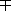\\db double bond \\square square \\tb triple bond \\neq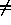\\ddb delocalized double bond \\rangle\\sim\\langle(N.B. ~  is the code for subscript) \\rightarrow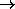\\simeq\\leftarrow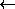\\inftyNote that \\db, \\tb and \\ddb should always be followed by a space, e.g. C=C is denoted by C\\db C.

### Special and virtual issues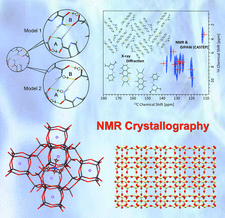Acta Crystallographica Section C has recently published special issues on

NMR Crystallography (March 2017)

Scorpionates: a golden anniversary (November 2016)

Full details are available on the special issues page.

The latest virtual issue features Coordination polymers, with an introduction by Len Barbour.

What are the 'most read' articles from the recent special issues?